# 2. (25 points) Two concentric spheres have radii Ri and R2 (R2 > Ri). The potential...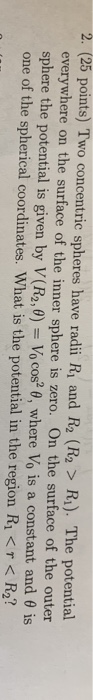2. (25 points) Two concentric spheres have radii Ri and R2 (R2 > Ri). The potential everywhere on the surface of the inner sphere is zero. On the surface of the outer sphere the potential is given by V(R2,0-10 cos2 θ, where Vo is a constant and θ is one of the spherical coordinates. What is the potential in the region Ri < R2?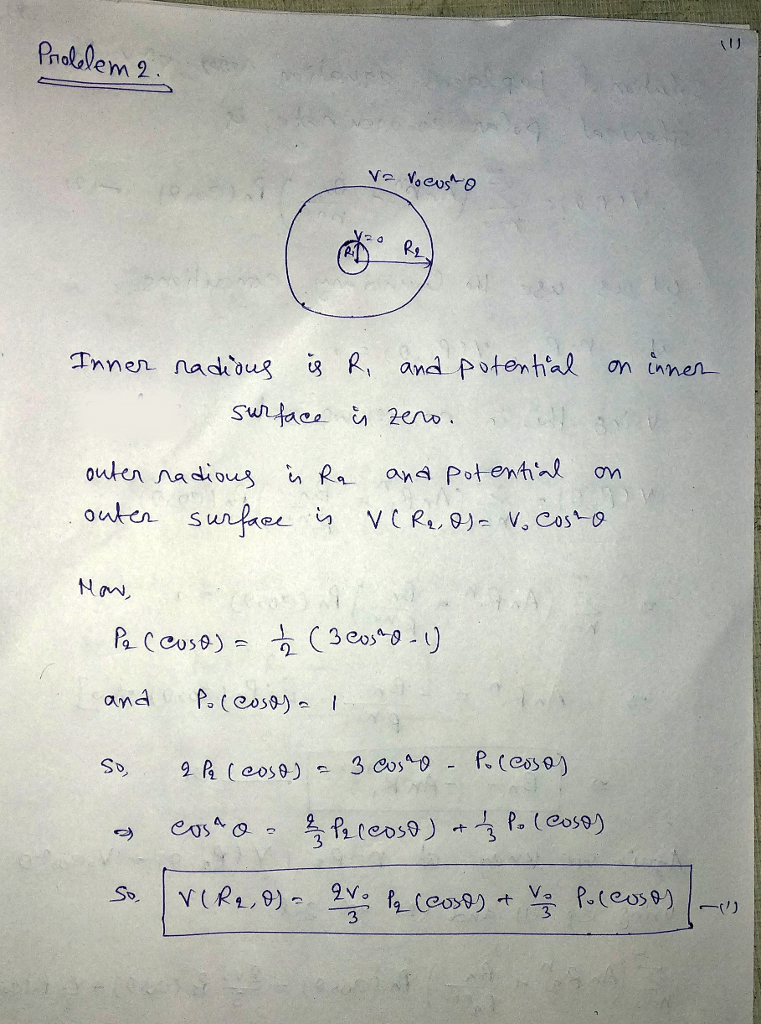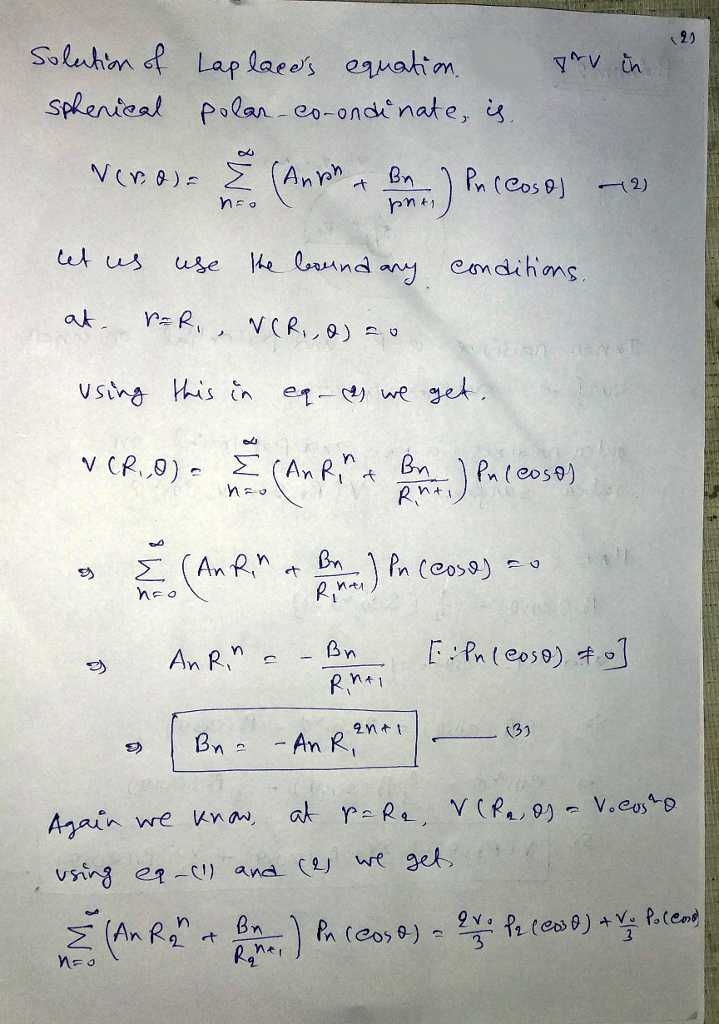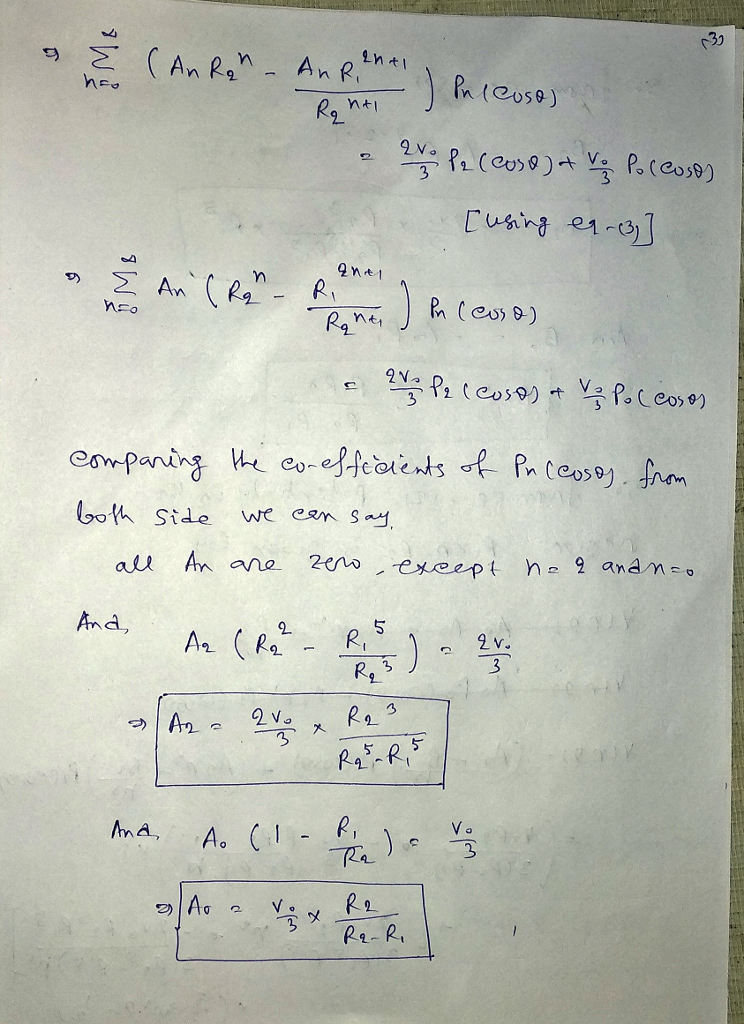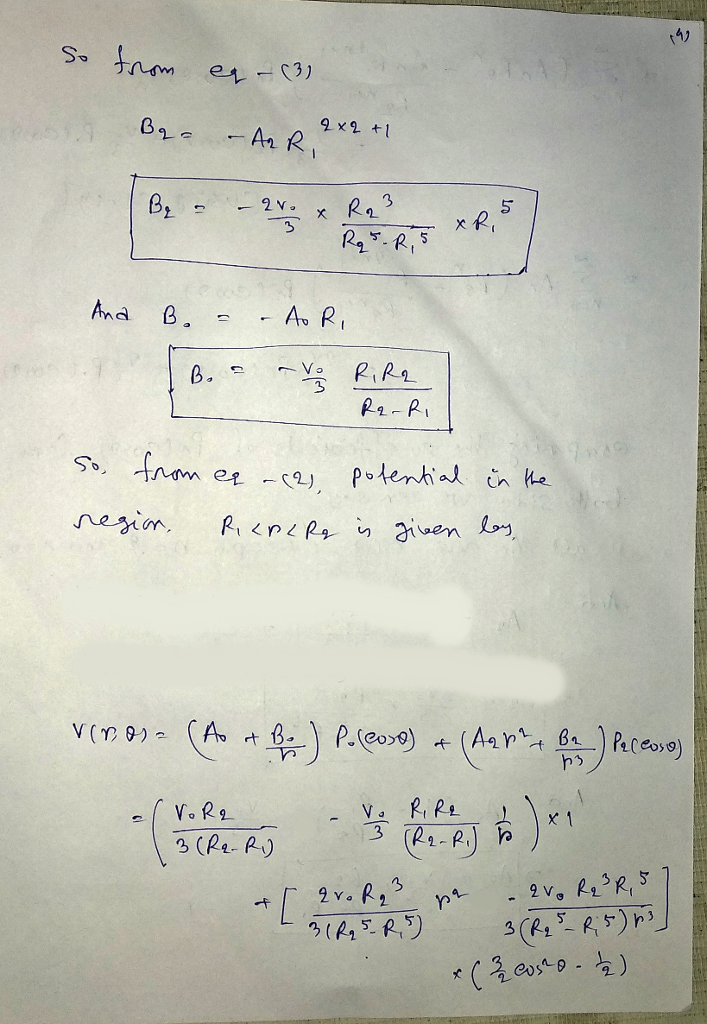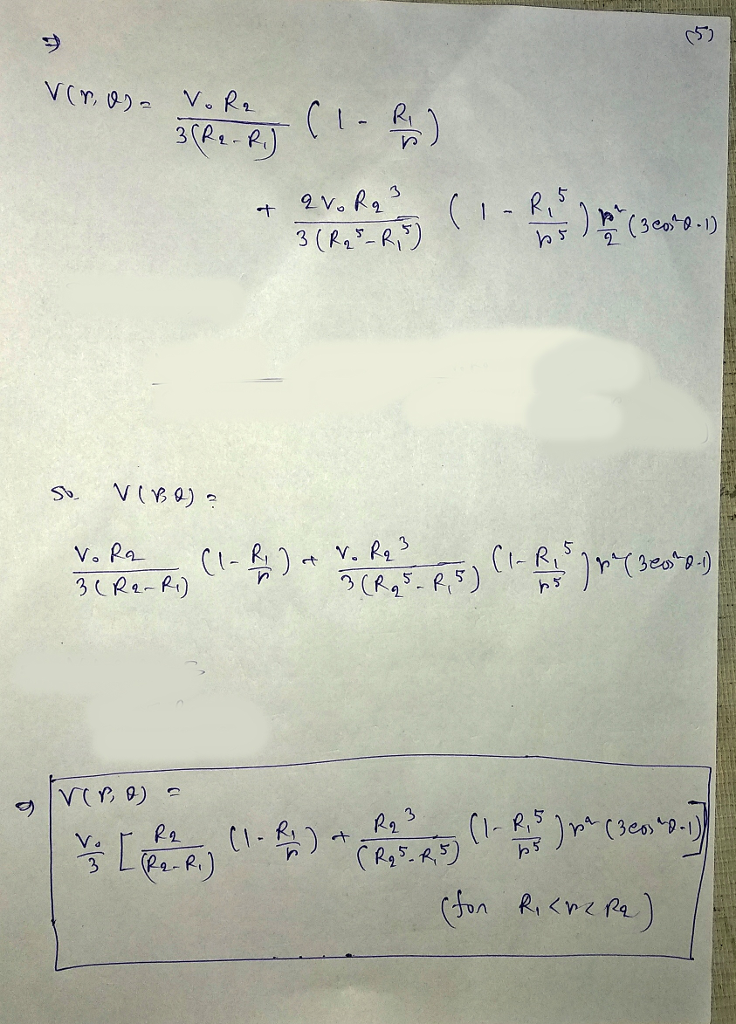#### Earn Coin

Coins can be redeemed for fabulous gifts.

Similar Homework Help Questions
• ### A total charge Q is uniformly distributed over the surface of two concentric con- ductive spheres of radii Ri R2 with t...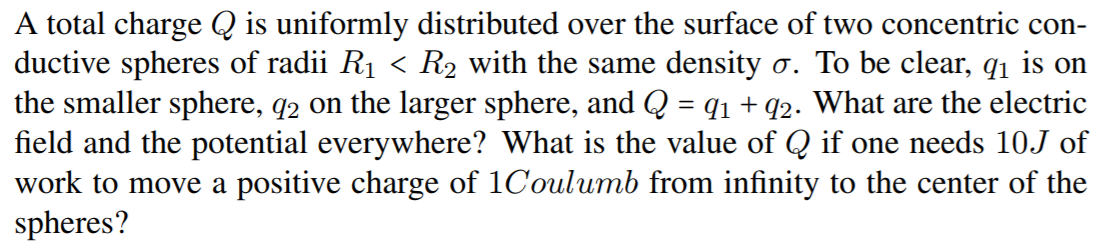A total charge Q is uniformly distributed over the surface of two concentric con- ductive spheres of radii Ri R2 with the same density σ. To be clear, qi is on the smaller sphere, g2 on the larger sphere, and Q2. What are the electric field and the potential everywhere? What is the value of Q if one needs 10.J of work to move a positive charge of 1Coulumb from infinity to the center of the くHo spheres? A total...

• ### 3. Two concentric spheres have radii R and 3 R. The inner sphere as a potential 2V,P (cos0) and the outer sphere a potential 46V.Ps(cos0). Find the potential at all points between the two spheres...3. Two concentric spheres have radii R and 3 R. The inner sphere as a potential 2V,P (cos0) and the outer sphere a potential 46V.Ps(cos0). Find the potential at all points between the two spheres. Extra credit include a 3D graph of the potential. 3. Two concentric spheres have radii R and 3 R. The inner sphere as a potential 2V,P (cos0) and the outer sphere a potential 46V.Ps(cos0). Find the potential at all points between the two spheres. Extra...

• ### 4. The accompanying ve shows two concentric spherical shells of negligible thickness and Radii Ri and...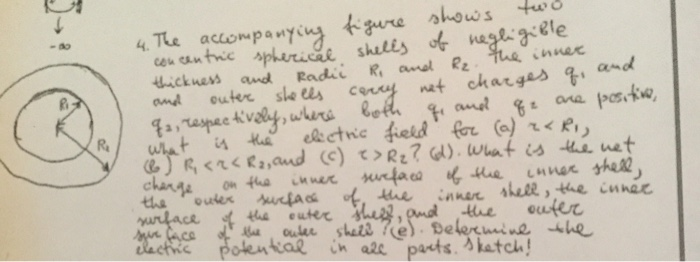4. The accompanying ve shows two concentric spherical shells of negligible thickness and Radii Ri and R₂. the inner and outer shells carry not charges q, and qa, respectively, where both qe and qe are positive, What is the electric field for (a) rari, 6 RC2CR2, and (c) ?> R2? (d). What is the net charge on the inner surface of the inner shell, the outer surface of the inner shell, the surface inner of the outer shes, and the...

• ### Use Gauss’ Law to solve for the electric field everywhere. Two conducting spheres concentric spheres of...

Use Gauss’ Law to solve for the electric field everywhere. Two conducting spheres concentric spheres of different radii (e.g. r1 and r2). The inner sphere has charge –Q and the outer charge has charge +2Q.

• ### 3.1 Two concentric spheres have radii a, b (b > a) and each is divided into...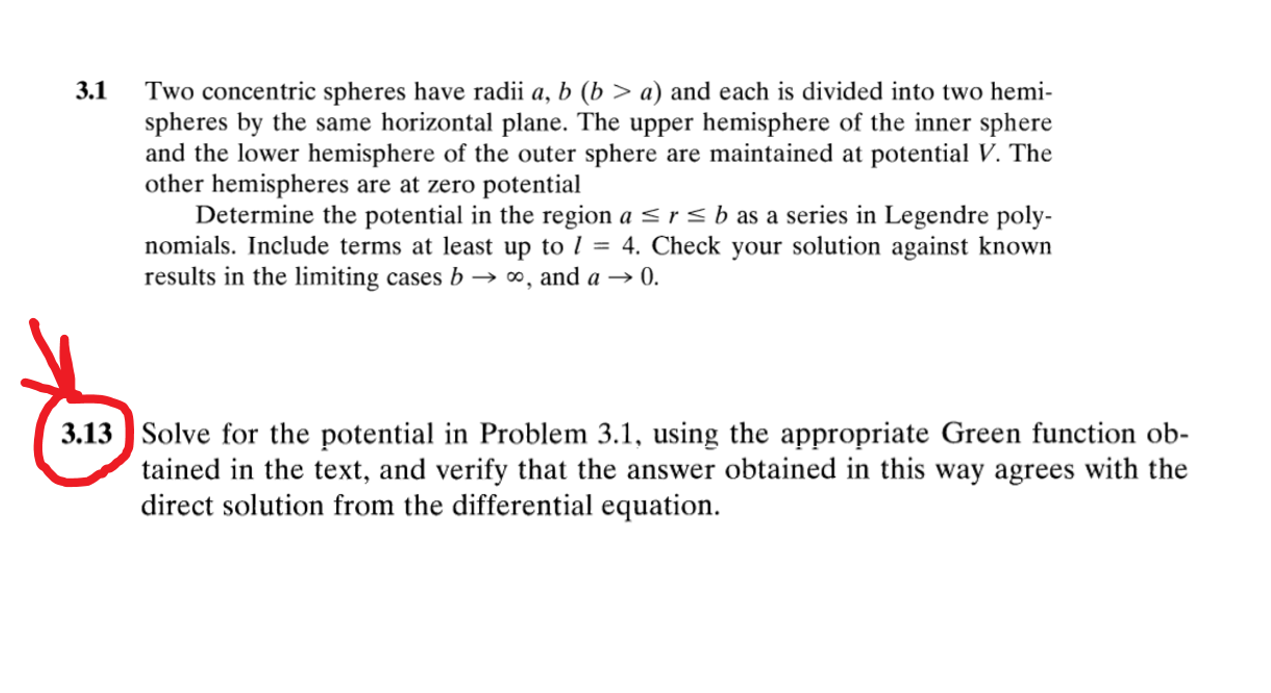3.1 Two concentric spheres have radii a, b (b > a) and each is divided into two hemi- spheres by the same horizontal plane. The upper hemisphere of the inner sphere and the lower hemisphere of the outer sphere are maintained at potential V. The other hemispheres are at zero potential Determine the potential in the region a <r < b as a series in Legendre poly- nomials. Include terms at least up to 1 = 4. Check your solution...

• ### Q#2: Consider two concentric conducting spheres of finite thickness in vacuum. The inner sphere has radii a, a, and car...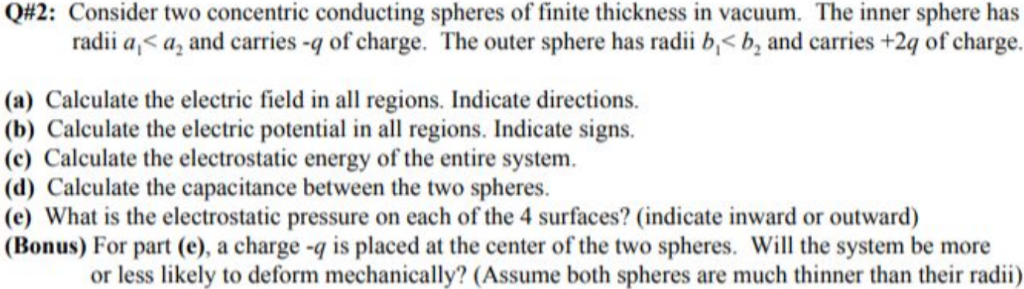Q#2: Consider two concentric conducting spheres of finite thickness in vacuum. The inner sphere has radii a, a, and carries -q of charge. The outer sphere has radii b,< b, and carries +2q of charge. (a) Calculate the electric field in all regions. Indicate directions (b) Calculate the electric potential in all regions. Indicate signs. (c) Calculate the electrostatic energy of the entire system (d) Calculate the capacitance between the two spheres. (e) What is the electrostatic pressure on each...

• ### (25) Two concentric spherical shells have radii rı = 5.00 cm and r2 = 10.0 cm....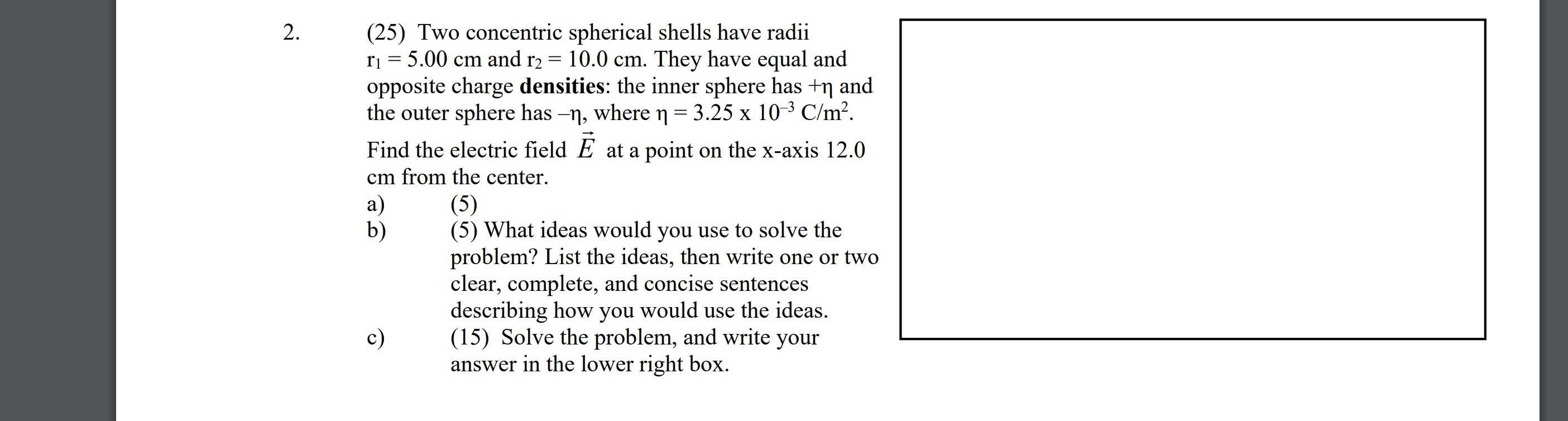(25) Two concentric spherical shells have radii rı = 5.00 cm and r2 = 10.0 cm. They have equal and opposite charge densities: the inner sphere has +ŋ and the outer sphere has –n, where n = 3.25 x 10-3 C/m². Find the electric field E at a point on the x-axis 12.0 cm from the center. (5) b) (5) What ideas would you use to solve the problem? List the ideas, then write one or two clear, complete, and...

• ### 3. Two axis aligned very long,thin shell cylinders have radii Ri and R2 with Ri 〈...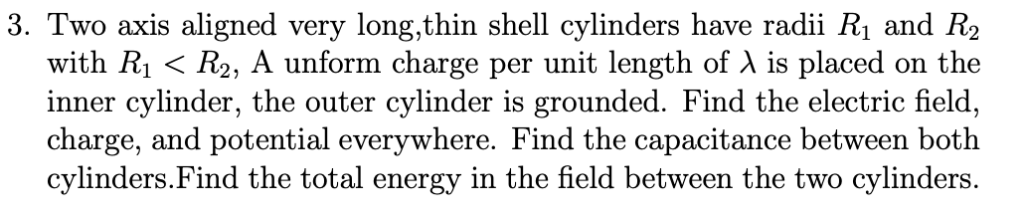3. Two axis aligned very long,thin shell cylinders have radii Ri and R2 with Ri 〈 R2, A unform charge per unit length of λ is placed on the inner cylinder, the outer cylinder is grounded. Find the electric field, charge, and potential everywhere. Find the capacitance between both cylinders.Find the total energy in the field between the two cylinders.

• ### ​Two concentric spheres are shown in the figure below.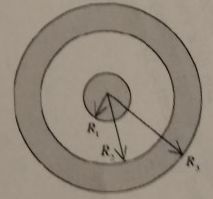Two concentric spheres are shown in the figure below. The inner sphere is a solid conductor and carries a charge of +5.00 μC uniformly distributed over its outer surface. The outer sphere is a conducting shell that carries a net charge of -8.00 μC. No other charges are present. The radii shown in the figure have the values R1 = 10.0cm, R2 = 20.0cm, and R3 = 30.0 cm. (a) (10 points) Find the total excess charge on the inner and...

• ### Flag question The outer sphere of two concentric conducting spheres of radii 9 cm and 15...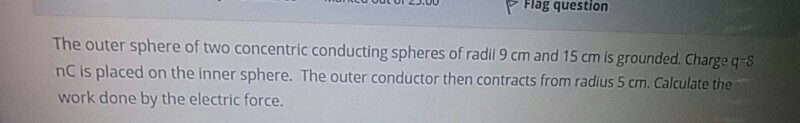Flag question The outer sphere of two concentric conducting spheres of radii 9 cm and 15 cm is grounded. Charge q=8 nC is placed on the inner sphere. The outer conductor then contracts from radius 5 cm. Calculate the work done by the electric force.